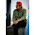## Search This Blog

% Find the root of y=cos(x) from o to pi.

f = @(x) (cos(x));
a = input('Please enter lower limit, a:  ');
b = input('Please enter upper limit, b:  ');
n = input('Please enter no. of iterations, n: ');
tol = input('Please enter tolerance, tol:  ');

fa = f(a); fb = f(b);
i = 1;

while i <= n
c = (b - a) / 2.0;
p = a + c;
fp = f(p);
if abs(fp) < 1.0e-20 | c < tol
fprintf('\nApproximate solution p = %11.8f \n \n',p);
break;
else
i = i+1;
if fa*fp > 0
a = p;
fa = fp;
else
b = p;
fb = fp;
end
end
end

>> bisection_method_md
Please enter lower limit, a:  0
Please enter upper limit, b:  pi
Please enter no. of iterations, n: 30
1.MATLAB